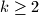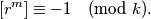### IMO Shortlist 1987 problem 23

Kvaliteta:
Avg: 0,0
Težina:
Avg: 0,0
Prove that for every natural number$k$ ($k \geq 2$) there exists an irrational number$r$ such that for every natural number$m$,Remark. An easier variant: Find$r$ as a root of a polynomial of second degree with integer coefficients.

Proposed by Yugoslavia.
Izvor: Međunarodna matematička olimpijada, shortlist 1987